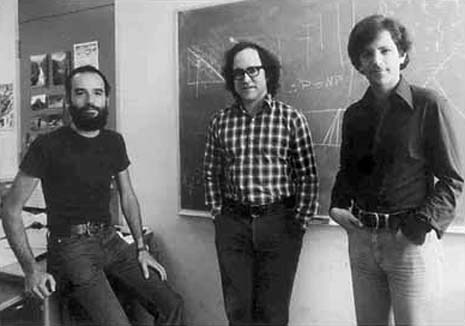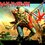# Introduction to cryptography(RSA Cipher)

In this note I want to discuss some ideas from modern cryptography..SuperComputer

Don't be intimidated by the size of the note...if you understand the ideas properly trust me it's worth it...you will learn about one of the most effective cipher systems still actively used today!!

$\textbf{Public-Key Cryptography:}$In conventional cryptographic systems like the Caesar cipher,the sender and the receiver jointly have a secret key that is used for encryption and decryption.But in public-key cryptography two keys are used - an encryption key and a decryption key.Though these keys are related,there is no easy way to derive the decryption key from the encryption key.Thus the encryption key can be made public without compromising the decryption key.A major advantage of the public cryptosystem is that it is unnecessary for the senders and the receivers to exchange a key in advance of their decision to communicate with one another.

$\textbf{Rivest-Shamir-Adleman Cipher:}$In 1977,Ronald Rivest,Adi Shamir and Leonhard Adleman proposed a public-key encryption system that uses elementary ideas from number theory.It's security depends on the assumption that in the current state of computer technology,factorization of composite numbers having large prime factors is so time consuming that it is almost unrealistic.RSA

$\textbf{Procedure:}$At first the user of the RS system chooses a pair of very large distinct primes.Then the two numbers are multiplied to form a number $n = pq$ called the $\text{enciphering modulus}$.The factorization of this product is beyond all computational abilities.Next,they need to select a positive integer $k$ such that $\gcd(k,\phi (n))=1$ called the $\text{enciphering exponent}$.the pair $(n,k)$ is kept in a public profile(like a telephone directory) as the user's personal encryption key which allows anyone else to encrypt and send a message to this individual,but the primes $p$ and $q$ are unknown to everybody else.prime

$\textbf{ALGORITHM:}$

$\textbf{Encryption:}$

$\textbf{Step 1.}$First each alphabet of the message to be sent is converted into a 2-digit number.A standard procedure for executing this is using the following assignment:ascii

$\textbf{Step 2.}$It is aasumed that the message $M < n$, where n is the enciphering modulus.Otherwise it is diffucult to distinguish M from any larger integer congruent to $M$ modulo n. If the message M is too long then it is broken into separate blocks of digits $M_1,M_2,....M_s$ of appropriate size and each is coded separately.

$\textbf{Step 3.}$After looking up the intended recipient's encryption key $(n,k$ in the public directory,the sender disguises the plaintext $M$ into the ciphertext $r$,by raising M to the $k$-th power and then taking modulo $n$,that is, $M^{k} \equiv r \pmod{n}$. $k$ is selected such that $gcd(k,\phi(n))=1$

$\textbf{Decryption:}$

$\textbf{Step 1.}$The authorized personnel deciphers the message by first determining the recovery exponent $j$, for which $kj \equiv 1 \pmod{\phi(n)}$

Since $gcd(k,\phi(n)) = 1$,this linear congruence has a uniqee solution modulo $\phi(n)$.The Euclidean Algorithm produces $j$ as a solution $x$ to the equation $kx+\phi(n)y = 1$. Note that the recovery exponent can only be obtained by someone who knows both $k$ and $\phi(n) = (p-1)(q-1)$ and hence both $p$ and $q$.The knowledge of any unauthorized third party is limited to the public key $(n,k)$.

$\textbf{Step 2.}$Now if we calculate $r^{j} \mod n$, we get,$\ r^{j} \equiv (M^{k})^{j} \equiv M^{1+\phi(n)*t} \equiv M*M^{\phi(n)*t}$ $\equiv M*1^{t} \equiv M \pmod n$ whenever $gcd(M,n)=1$.

In other words raising the plaintext number to the $j$-th power and taking moduulo n recovers the plaintext.

It is to be noted that the assumption $gcd(M,n)=1$ was used to use Euler's theorem.In case they are not relatively prime, we can prove by similar argument that $r^{j} \equiv M \pmod p$ $r^{j} \equiv M \pmod q$ Since $gcd(p,q)=1$, we have $r^{j} \equiv M \pmod{pq}$,that is $r^{j} \equiv M \pmod n$!!

$\textbf{Step3.}$Convert the digital representation to the alphabetic one.

$\textbf{Example:}$

Let us consider an unrealistically small example choosing 2 primes $p = 29$ and $q=53$.

The enciphering modulus is then $n = 29*53 = 1537$ and $\phi(n) = 28*52 = 1456$.

Since $gcd(47,1436)=1$, we may choose $k=47$ to be the enciphering exponent.It can be calculated that in this case the recovery exponent will be $31$.

Let the first block from the digital version of the plaintext be $131$,this encrypts to the ciphertext $131^{47} \equiv 570 \pmod{1537}$.But by using the recovery exponent $j = 31$, we can decrypt this to $570^{31} \equiv 131 \pmod{1537}$.

This is the original digital form of the plaintext !!!

$\textbf{Applications:}$Banks(1024-bit),Facebook(1024-bit),Twitter(1024-bit),Ebay(1024-bit) all use the RSA cipher to protect our internet secrets!!The numbers in the brackets denote the number of digits of the enciphering modulus. A 768 bit key has recently been broken.hack

Have fun encrypting :D ....i'll try to do a few more posts on modern ciphers next week

$\textbf{References:}$

$1.$Introduction to Algorithms by Cormen,Leiserson,Rivest and Stein

$2.$Elementary Number Theory by David M.Burton(I took the example from here)

$3.$Encryption and huge numbers by NumberPhile (Video by Brady Haran and demonstrated by Dr.James Grime)6 years ago

This discussion board is a place to discuss our Daily Challenges and the math and science related to those challenges. Explanations are more than just a solution — they should explain the steps and thinking strategies that you used to obtain the solution. Comments should further the discussion of math and science.

When posting on Brilliant:

• Use the emojis to react to an explanation, whether you're congratulating a job well done , or just really confused .
• Ask specific questions about the challenge or the steps in somebody's explanation. Well-posed questions can add a lot to the discussion, but posting "I don't understand!" doesn't help anyone.
• Try to contribute something new to the discussion, whether it is an extension, generalization or other idea related to the challenge.

MarkdownAppears as
*italics* or _italics_ italics
**bold** or __bold__ bold
- bulleted- list
• bulleted
• list
1. numbered2. list
1. numbered
2. list
Note: you must add a full line of space before and after lists for them to show up correctly
paragraph 1paragraph 2

paragraph 1

paragraph 2

[example link](https://brilliant.org)example link
> This is a quote
This is a quote
    # I indented these lines
# 4 spaces, and now they show
# up as a code block.

print "hello world"
# I indented these lines
# 4 spaces, and now they show
# up as a code block.

print "hello world"
MathAppears as
Remember to wrap math in $$ ... $$ or $ ... $ to ensure proper formatting.
2 \times 3 $2 \times 3$
2^{34} $2^{34}$
a_{i-1} $a_{i-1}$
\frac{2}{3} $\frac{2}{3}$
\sqrt{2} $\sqrt{2}$
\sum_{i=1}^3 $\sum_{i=1}^3$
\sin \theta $\sin \theta$
\boxed{123} $\boxed{123}$

Sort by:

Well i always was in need of learnin about this!!.....NOW it;s HERE! thnx "Eddie the Head" i might need to contact u for discussions...if u agree?.see my brilliant profile..

- 5 years, 10 months ago

Sure...I'm always available for discussion... also check out my other cryptography posts :)

- 5 years, 10 months ago

This is amazing! I loved reading this!

- 6 years ago

Thanks! I hope you won't mind if I post another link here which contains some basic knowledge on Cryptography (it might help someone out) : Khan Academy Videos on Cryptography.

- 6 years ago

You re welcome to post any related material:)

- 5 years, 12 months ago

gr8 stuff ..........

Wow, interesting stuff! Who would've imagined how far a supposedly minor theorem such as Fermat's Little Theorem could go?

- 6 years ago